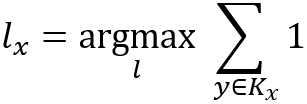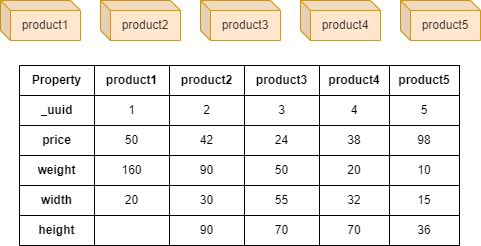# Change Nickname

Current Nickname:
Search
v4.0
v4.0

# k-Nearest Neighbor

## Overview

k-Nearest Neighbor (kNN) algorithm, also known as the nearest neighbor algorithm, classifies a given sample based on the classifications of its K most similar (e.g. Cosine Similarity) samples. Proposed in 1967 by IEEE members T. M. Cover and P. E. HART, this algorithm is one of the simplest data classification techniques.

Although contains the word 'neighbor' in its name, kNN does not rely on the relationship between samples when calculating similar samples, in graph it means that kNN has nothing to do with the edges between nodes.

Related material of the algorithm:

## Basic Concept

### Select Similar Nodes

Ultipa's kNN algorithm defines the sample as node in the graph and uses cosine similarity (read the chapter Cosine Similarity) between nodes as the similarity between the samples, then to select K nodes `y` that are most similar to the given node `x` as the K nearest neighbors `Kx`.

### Vote on Classification

Using one node property as the classification label, and count the labels of the selected K nearest neighbors, and the label with the most occurrences will be used as the label of `x`:If there are more than one labels that appear the most, the label of the node which has the highest similarity will be used.

## Special Case

### Lonely Node, Disconnected Graph

Theoretically, the calculation of kNN does not depend on the existence of edges in the graph, and regardless of whether the node to be calculated is lonely node or which connected component it locates, it does not affect the calculation of kNN.

### Self-loop Edge

The calculation of kNN has nothing to do with edges.

### Directed Edge

The calculation of kNN has nothing to do with edges.

## Results and Statistics

The graph below has 5 product nodes (edges are ignored), product node has properties price, weight, weight and height:Algorithm results: Given node product1, select the most similar 3 nodes based on properties price, weight and width, and specify property height as the classification label

• The voted classification label `attribute_value` and the number of times `count` it appears in the 3 kNN
attribute_value count
70 2
• The selected 3 kNN `node` and its cosine similarity `similarity` between product1
node similarity
4 0.6348920499152856
3 0.7811137489489366
2 0.9763079726833555

Note: The cosine similarity between product5 and product1 is 0.404234826778782, thus it is not involved in the 3 kNN.

Algorithm statistics: N/A

## Command and Configuration

• Command: `algo(knn)`
• Configurations for the parameter `params()`:
Name
Type
Default
Specification Description
node_id `_uuid` / Mandatory UUID of the node to be calculated
node_schema_property []`@<schema>?.<property>` / Numeric node property, LTE needed; at least two properties are required, the schema should be same with the schema of node node_id Properties of the node that participate in the calculation of cosine similarity
top_k int / >0, mandatory The number of the most similar nodes to select and vote on the classification label
target_schema_property `@<schema>?.<property>` / LTE needed, and the schema should be same with the schema of node node_id The property of node where the classification label is located

Example: Estimate the value of height according to the property price, weight and width of node UUID = 1

``````algo(knn).params({
node_id: 1,
node_schema_property: [@product.price,@product.weight,@product.width],
top_k: 4,
target_schema_property: @product.height
}) as height return height
``````

## Algorithm Execution

#### 1. File Writeback

Configuration
Data in Each Row Description
filename First row: `attribute_value`, second row and later: `_id`,`similarity` First row is the elected classification label, second row and later are the selected kNN node ID and its cosine similarity between the given node

Example: Estimate the value of height according to property price, weight and width of node UUID = 1, write the algorithm results back to file named knn

``````algo(knn).params({
node_id: 1,
node_schema_property: [@product.price,@product.weight,@product.width],
top_k: 4,
target_schema_property: @product.height
}).write({
file:{
filename: "knn"
}
})
``````

#### 2. Property Writeback

Not supported by this algorithm.

#### 3. Statistics Writeback

Not supported by this algorithm.

### Direct Return

Alias Ordinal
Type
Description Column Name
0 KV The elected classification label and the number of occurrences it appears in K nearest neighbors `attribute_value`, `count`
1 []perNode The selected K nearest neighbors and their cosine similarities `node`, `similarity`

Example: Estimate the value of height according to property price, weight and width of node UUID = 1, define algorithm results as alias named a1 and a2 and return the results

``````algo(knn).params({
node_id: 1,
node_schema_property: [@product.price,@product.weight,@product.width],
top_k: 3,
target_schema_property: @product.height
}) as a1, a2 return a1, a2
``````

### Streaming Return

Alias Ordinal
Type
Description Column Name
0 KV The elected classification label and the number of occurrences it appears in K nearest neighbors `attribute_value`, `count`

Example: Estimate the value of height according to property price, weight and width of node UUID = 1, define algorithm results as alias named a1 and return the results

``````algo(knn).params({
node_id: 1,
node_schema_property: [@product.price,@product.weight,@product.width],
top_k: 3,
target_schema_property: @product.height
}) as a1 return a1
``````

### Real-time Statistics

This algorithm has no statistics.